Next: Theoretical Foundation - Takens' Up: Geometry from an Ideal Previous: Geometry from an Ideal   Contents

## The Principle of Reconstruction - Method of Delays

The first group to propose a solution for the problem of extracting geometric information from time series was Packard et al. . The basic idea of their approach is that the state of an-dimensional dynamical system can be uniquely characterized byindependent quantities. One such set of independent quantities are, of course, the phase space coordinates (more exact: the coordinates in some-dimensional basis which spans
)
(8)

but these are not available, since the only data one has is the one-dimensional time series. Based on the conjecture that any-tupel of numbers should give equivalent results (in the sense that, if one reconstructs several phase portraits in accordance with this idea, then for any two of these phase portraits there should be a diffeomorphism which maps one onto the other) Packard et al. proposed two different possibilities to construct-vectors which in some sense contain the same information as the original state vectors in eq. (8): One can work with the method of delays which simply takesconsecutive elements of the time series directly as coordinates in phase space:(9)

For example when analyzing a dynamical system it is often convenient just to take one coordinate of phase space as the observable:(10)

The other possibility suggested by Packard et al. is to use the time series to construct time derivatives of the measured observable2 and use the vectors
(11)

In this way the one-dimensional time series is used to build a sequence of-dimensional vectors which form a trajectory in some-dimensional space. Numerical experiments done by Packard et al. show that this procedure gives reasonable results: the phase-space pictures one gets seem closely related to the corresponding pictures of the original dynamical system. In particular, topological properties and the geometrical form of the attractor seem to be conserved under this procedure. As an illustration of this method of phase space reconstruction, we present a result of Broomhead and King : They considered the Lorenz system:
(12)
(with the parameter values
,,
). First they integrated this set of ordinary differential equations to get the complete solution''; the projection of this solution onto the-plane is shown in Fig. 1.a. They chose the
-coordinate to be their observable''and thus got the one-dimensional data stream as shown in Fig. 1.b. Then, after choosing some delay time
, they obtained a discrete time series from the data stream and used this time series to construct a phase portrait, using the method of delays: See Fig. 1.c. Comparison of the original Lorenz attractor (Fig. 1.a) and the reconstructed one justifies Packard's approach: Although the pictures are not exactly the same and although Fig. 1.a is much coarser than Fig. 1.c, both of them seem to show an object with the same geometry. A shortcoming of Packard's method is that it does not give a general strategy how to choose. If one knows that the dynamical system is evolving in
-dimensional phase space then one can use the idea mentioned above and take
. But if one is analyzing a system about the dimensionality of which nothing is known a priori then how to proceed? This problem will be addressed in section 3. It is also not quite clear whatshould be when we try to reconstruct phase portrait of a system with infinitely many degrees of freedom, such as the Mackey-Glass equation ([5,12] and , chapter 5.3):(13)
#### Footnotes

... observable2
One can compute an approximation to the derivatives from the time series for example by using the formula
where theare determined by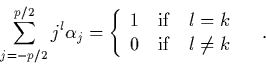This formula results from differentiating the interpolating polynomial. See , §§ 27 and 28, for details of this method of numerical differentiation. See , chapter 3.4, for a more sophisticated extrapolation method.Next: Theoretical Foundation - Takens' Up: Geometry from an Ideal Previous: Geometry from an Ideal   Contents
Martin_Engel 2000-05-25